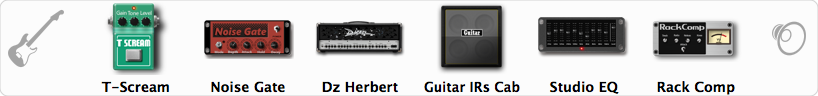# Diezel Metal

Discussion in 'ToneLib-GFX presets' started by Diego Castro, May 17, 2019.

1. Diezel Metal

GFX version: 3.0

Preset name: DieselMetal
Optimized for: Phones/Speaker

Effects chain:Archtects style high gain tone

Effect: "T-Scream" (Overdrive / Distortion), active - "yes"
"Drive" = 26
"Tone" = 50
"Level" = 79

Effect: "Noise Gate" (Dynamics / Filter), active - "yes"
"Detect" = Gtr In
"Mode" = Manual
"Depth" = 100
"Threshold" = 68
"Attack" = 0
"Hold" = 5
"Decay" = 24

Effect: "Dz Herbert" (Amp simulators), active - "yes"
"Gain" = 84
"Bass" = 31
"Middle" = 46
"Treble" = 65
"Presence" = 62
"Master" = 58
"Output" = 50
"Level (dB)" = 0

Effect: "Guitar IRs Cab" (Cabinets), active - "yes"
"Model" = Marshall 1960A (4x12")
"Mic Position" = Center
"Mic Distance" = Near
"Low Cut (Hz)" = 60
"Hi Cut (kHz)" = 10.5
"Mix" = 100
"Level (dB)" = 0

Effect: "Studio EQ" (Dynamics / Filter), active - "yes"
"31 Hz" = 0
"62 Hz" = 0
"125 Hz" = -3
"250 Hz" = -2
"500 Hz" = 0
"1 kHz" = -1
"2 kHz" = 0
"4 kHz" = 0
"8 kHz" = -1
"16 kHz" = 0
"above 16 kHz" = 0
"Level (dB)" = 0

Effect: "Rack Comp" (Dynamics / Filter), active - "yes"
"Threshold (dB)" = -8
"Ratio" = 4
"Attack" = Fast
"Release (ms)" = 141
"Knee" = 0
"Level (dB)" = 0

Note: This is a ToneLib-GFX preset file, you will need to download and install the ToneLib-GFX software to use the preset.

File size:
624 bytes
Views:
5,534